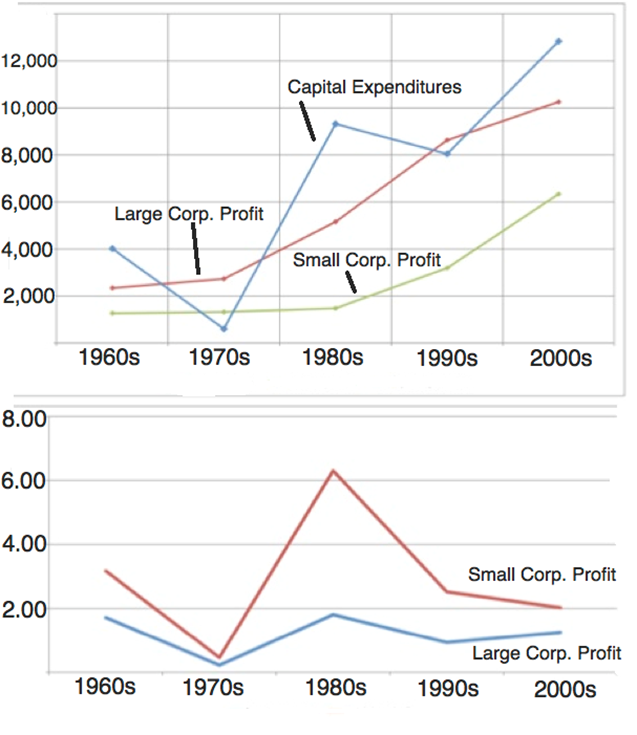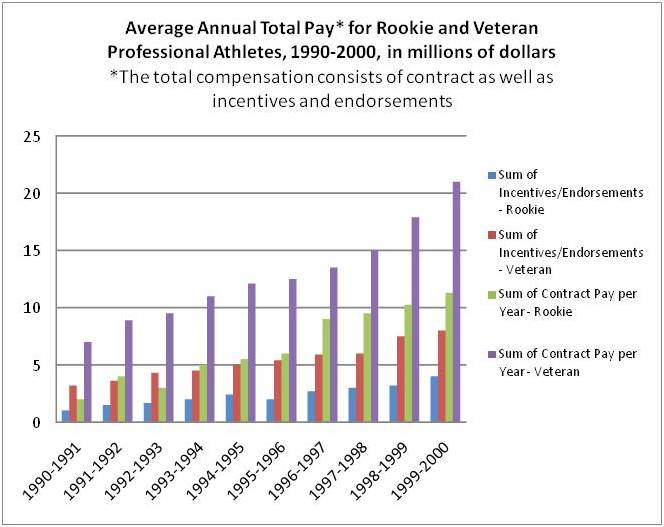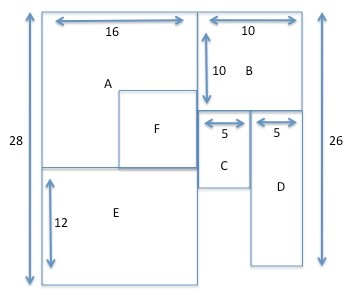+91-22-62437100 / 01 | help@reachivy.com

# GRE Multiple-choice Quiz 2

One of the question types in GRE is multiple-choice questions in which you are asked to select only one answer choice from a list of five choices. To understand what multiple choice questions are, attempt the quiz below.

To begin click Next

Question 1:

Mr. McCall selects a number that is two-digit and positive. If the number is prime, he assigns that many problems for homework. If the number is not prime, he assigns 8 more problems than the number for homework. If he assigns 97 problems for homework, what is the number?
I. 89
II. 97
III. 105

Question 2:

Which of the following numbers has the greatest number of unique digits?
Question 3:

A delivery cart went from Candleford to Lark Rise and back at an average speed of 2/3 miles per hour. If the distance from Candleford to Lark Rise is 1 mile, and the trip back took half as much time as the trip there, what was the average speed of the delivery cart on the way to Lark Rise?

Question 4:

The midpoints of the sides of a square are connected to form a new inscribed square. How many times greater than the area of the inscribed square is the area of the original square?

Question 5:

The diameter of a circle is increased by 80%. By what percent, approximately, is the area increased?

Question 6:By approximately what percent did the large corporate profits increase from the 1960s to the 2000s?

Question 7:In what year shown was the total annual pay for veterans closest to \$15 million?

Question 8:

The figure below is the landscaping plan for Ersne's new office park. The thickness of the dividing walls should be ignored and the dimensions are in feet.F = 1/4 A and C = 1/2 D
If the dividing walls between each specified area are going to be painted, how many square feet will be painted?

You have answered all the questions in the quiz. Press Submit to view your score